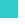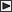BRI Research Paper

 No.96 Statistical Analysis of Fire Spread. Y.Morishita; May, 1982. 16p. Abstract In this paper, statistical techniques which can be applied to the estimation of fire spread are introduced, together with their application examples to Occurrance of Flashover in Fire Tests and Development of Real Fire in Dwellings. These techniques are called "Theory of Quantification", since numerical values are given to the factors expressed in nominal scale so as to estimate fire spread most precisely, based on the assumption of a linear sum model of the factors, where coefficients are given to the factors expressed in interval scale as well. Comparison of the effects of the factors can be achieved by comparing the ranges or the partial correlations calculated by using the given values to the factors. In the application example of these techniques to Fire Tests Data, the domain where flashover occurres are shown on the two dimensional Euclidian space using linear sum of the facters.Concerning Real Fire Data, the discrimination if a fire develops up to the phase that a room of fire origin is wholly involved is conducted. The development of such analysis of Fire Test Data or Real Fire Data which are accumulated so far will give more valuable and direct informations to the estimation of fire spread. * Research Member, Testing & Evaluation DepartmentLinksSitemapInquiryJapanese HP

BUILDING RESEARCH INSTITUTE, JAPAN